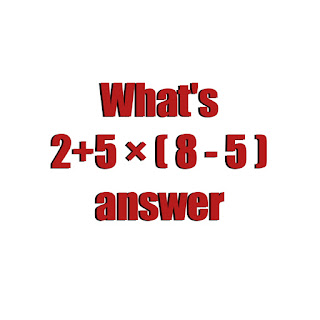# What's 2 + 5 × ( 8 - 5 ) math Quiz answer

0

What's 2 + 5 × ( 8 - 5 ) math Quiz answer

The solution to What is 2 + 5 × ( 8 - 5 )

Answer? is  simple but tricky. Many people always fall into the trap of not using basic mathematical principles and rules such as BODMAS and PEMDAS .  This Quiz is trending online special on Facebook and YouTube confusing may people.

Today I will solve this Math Quiz using

Bodmas. The correct answer would be 17 not 21.Check details belowWhat's 2 + 5 × ( 8 - 5 ) math Quiz answer

BODMAS stands for:

B=Bracket

O=Order of operations

D=Division

M=Multiplication

S=Subtraction.

Answer to  2 + 5 ×( 8 - 5 ) Math Quiz

I will solve what is in the bracket first.

8-5= 3

Implies 2 + 5 × ( 8 - 5 ) =2 + 5 ×( 3 )

2 + 5 × 3

2 + 15

17

Therefore the answer to 2 + 5 × ( 8 - 5 ) is 17.

Share to friends and family

Recommendation for you:

What's 50 + 10 × 0 + 7 + 2 = ? Math Quiz answer

Tags